# Waveform RMS and Average Values

Sine Wave
Full Wave Rectified
Half Wave Rectified
AC Superposed on DC
Periodic Half Sinusoids
Square Wave
Sawtooth Wave
Trapezoidal Wave

The standard formula for calculating the RMS (Root Mean Square) values for a waveform, I(t), is:

The tables below show equations for calculating the typical waveform RMS and average values.

 Current Wave Shape Configuration IRMS IAVGDiagram for a Sine Wave RMS Calculation Sine Wave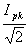0

 Current Wave Shape Configuration IRMS IAVGDiagram for a (Full Wave) Rectified Sine Wave RMS Calculation Full Wave Rectified Sine Wave0.637Ipk

 Current Wave Shape Configuration IRMS IAVGDiagram for a Half Wave Rectified Sine Wave RMS Calculation Half Wave Rectified Sine Wave0.318Ipk

 Current Wave Shape Configuration IRMS IAVGDiagram for a Sine Wave With a DC Offset RMS Calculation Direct Current with Superposed Sine WaveIdc

 Current Wave Shape Configuration IRMS IAVGDiagram for a Repetitive Half Sinusoid Waveform RMS Calculation Half-Sinusoids of Duration T and Frequency f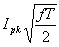(2fIpkT)/ p

 Current Wave Shape Configuration IRMS IAVG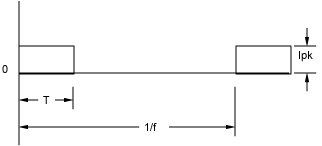Diagram for a Repetitive Square Wave RMS Calculation Square Waves of Duration T and Frequency f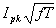fIpkT

 Current Wave Shape Configuration IRMS IAVGDiagram for a Repetitive Sawtooth Waveform RMS Calculation Sawtooth Waveform of Duration T and Frequency f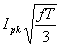0.5fIpkT

 Current Wave Shape Configuration IRMS IAVG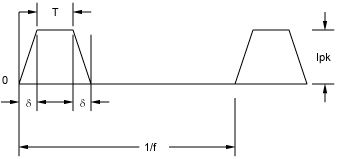Diagram for a Repetitive Trapezoidal Waveform RMS Calculation Trapezoidal Waveform of Frequency f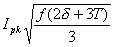fIpk( d+T)

Send consulting inquiries, comments, and suggestions to richard.ness@nessengr.com